﻿ 江西省气象-水文-雷达小时雨量信息融合试验结果分析
 期刊检索： 题目 作者 关键词
 暴雨灾害2020, Vol. 39Issue (3): 276-284.  DOI: 10.3969/j.issn.1004-9045.2020.03.008### DOI

10.3969/j.issn.1004-9045.2020.03.008

### 文章历史

Analysis of fusion test results on hourly precipitation from meteorological and hydrological stations and radar
LI Xianfeng, ZHANG Wei, HUANG Shaoping , DENG Weihua , ZHOU Xueying
Jiangxi Meteorological Information Center, Nanchang 330096
Abstract: Based on the precipitation data from the meteorological and hydrological stations and the Quantitative Precipitation Estimation (QPE) from radars over Jiangxi between May and June in 2019, we have made a fusion test of hourly precipitation from the meteorological and hydrological stations and by radars with 1 km×1 km resolution by using the space-time multiscale analysis system (STMAS), and made an analysis and assessment of fusion test results. The results show that the precipitation detected by meteorological stations, hydrological stations and radars has a similar spatial distribution. It is found that the severe precipitation zones and the patterns of precipitation distribution are very consistent. Comparing with the precipitation observed, the different fusion test results have a consistent variation tendency, but all are the underestimating to a certain extent. Those fused precipitation from the hydrological stations have a significant improvement in underestimation. The independent verification results indicate that the test results fused precipitation from hydrological stations have an obvious improvement in the accuracy of different level of precipitation, whose correlation coefficient increases by 9.2%, and root mean square error and mean absolute error decreases by 14.3% and 12.1%, respectively. After further fusing precipitation from hydrological stations, precipitation fusion results of different height areas are significantly improved, especially in the areas of elevation greater than 800 m whose correlation coefficient increases by 8.8% and root mean square error decreases by 14.1%.
Key words: hourly precipitation    fusion test    meteorological station    hydrological station    Quantitative Precipitation Estimation    space-time multiscale analysis system

1 数据预处理与融合方法 1.1 数据及预处理 1.1.1 气象站观测降水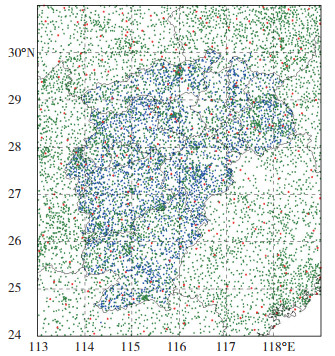图 1 研究区域国家级气象观测站(红点)、区域气象自动站(绿点)与水文站(蓝点)分布 Fig. 1 Spatial distribution of national weather stations (red points), regional automatic weather stations (green points) and hydrological stations (blue points) in this study area.
1.1.2 水文站观测降水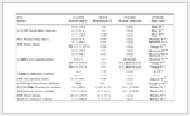表 1 江西省气象站与水文站的雨量观测设备配置情况 Table 1 Configuration of rainfall observation equipment displayed in the meteorological and hydrological stations over Jiangxi Province.

1.1.3 雷达估测降水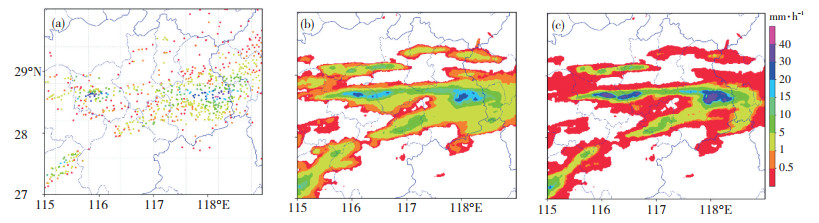图 2 2019年6月6日15时赣东北区域地面观测降水实况(a)以及订正前(b)、订正后(c)的雷达估测降水分布(单位: mm·h-1) Fig. 2 Spatial distribution of (a) surface precipitation observations (unit: mm·h-1) and radar estimate precipitation (unit: mm·h-1) (b) before and (c) after corrected in the northeast Jiangxi at 15:00 BT on 6 June 2019.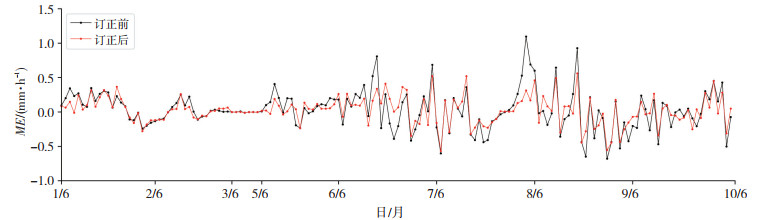图 3 2019年6月1—10日江西省逐小时雷达估测降水订正前(黑线)与订正后(红线)的平均误差(ME, 单位: mm·h-1)时间变化 Fig. 3 Hourly variation of mean error (unit: mm·h-1) of quantitative precipitation estimation from radar before (black line) and after (red line) corrected in Jiangxi from June 1 to 10 in 2019.
1.2 融合方法

 \begin{aligned} J^{(n)}=& \frac{1}{2} X^{(n) T} X^{(n)}+\frac{1}{2}\left(H^{(n)} X^{(n)}-\right.\\ &\left.Y^{(n)}\right)^{T} O^{(n)-1}\left(H^{(n)} X^{(n)}-Y^{(n)}\right) \end{aligned} (1)

 $\left\{\begin{array}{ll} Y^{(1)}=T^{\mathrm{obs}}-H^{(1)} X^{\mathrm{b}} & (n=1) \\ Y^{(n)}=Y^{(n-1)}-H^{(n-1)} X^{(n-1)} & (n=2, 3, \cdots, N) \end{array}\right.$ (2)

 $X^{\mathrm{a}}=X^{\mathrm{b}}+\Delta X=X^{\mathrm{b}}+\sum _{n=1}^{N} X^{(n)}$ (3)表 2 2019年5—6月江西省不同影响半径(r，网格数)融合试验的检验结果 Table 2 Evaluation of the fusion test results with the different influence radius (r, grid number) in Jiangxi between May and June in 2019.
1.3 融合试验表 3 考虑不同降水数据源的融合试验方案 Table 3 The fusion test schemes using different precipitation data source.
1.4 评价方法

 $C O R=\frac{\sum _{j=1}^{N}\left(G_{i}-\bar{G}\right)\left(O_{i}-\bar{O}\right)}{\sqrt{\sum _{j=1}^{N}\left(G_{i}-\bar{G}\right)^{2}} \sqrt{\sum _{i=1}^{N}\left(O_{i}-\bar{O}\right)^{2}}}$ (4)
 $R M S E=\sqrt{\frac{1}{N} \sqrt{\sum _{j=1}^{N}\left(G_{i}-O_{i}\right)^{2}}}$ (5)
 $M E=\frac{1}{N} \sum _{i=1}^{N}\left(G_{i}-O_{i}\right)$ (6)
 $M A E=\frac{1}{N} \sum _{i=1}^{N}\left(\left|G_{i}-O_{i}\right|\right)$ (7)
 \$ T S_{k}=\frac{\sum _{i=1}^{N}\left[G_{i} \geqslant L_{k}\right]\left[O_{i} \geqslant L_{k}\right]}{\sum _{i=1}^{N}\left(\left[G_{i} \geqslant L_{k}\right]+\left[G_{i}

2 结果与分析

2.1 空间分布对比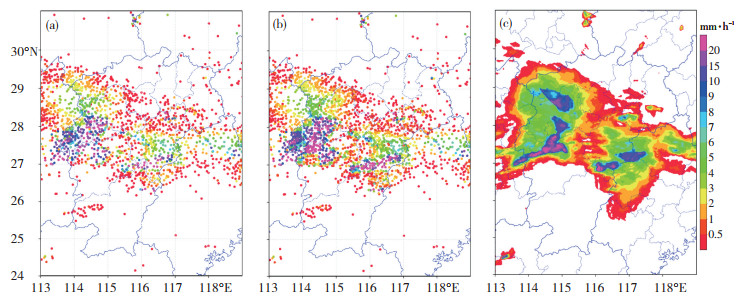图 4 2019年6月8日21时江西省气象站(a)、水文站(b)、雷达(c)观测降水(单位：mm·h-1)空间分布 Fig. 4 Spatial distribution of precipication (unit:mm·h-1) from (a) weather stations, (b) hydrological stations and (c) radar over Jiangxi at 21:00 BT on 8 June 2019.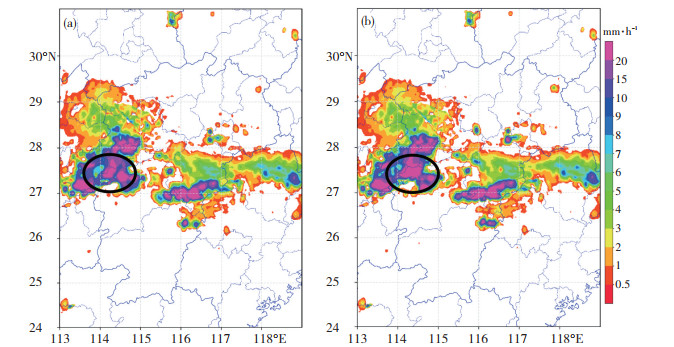图 5 由融合试验方案Ⅰ (a)、方案Ⅱ (b)获取的江西省2019年6月8日21时小时降水(单位: mm·h-1)分布(黑色线圈表示小时雨量大于15 mm·h-1的强降水区) Fig. 5 Hourly precipation (unit: mm·h-1) from (a) scheme Ⅰ and (b) scheme Ⅱ of fusion tests in Jiangxi at 21:00 BT on 8 June 2019. Black circles denote severe precipation areas with rainfall of being greater than 15 mm·h-1.
2.2 逐小时序列对比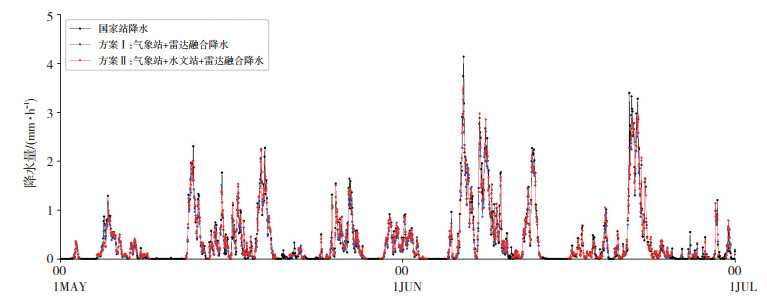图 6 2019年5月1日00时—6月30日23时方案Ⅰ (蓝线)、方案Ⅱ (红线)融合降水与江西省国家站(黑线)降水逐时变化(单位: mm·h-1) Fig. 6 Hourly variation of precipitation (unit: mm·h-1) from scheme Ⅰ (blue line) and scheme Ⅱ (red line) of fusion tests and the national weather stations over Jiangxi between 00:00 BT 1 May and 23:00 BT 30 June in 2019.
2.3 结果检验

2.3.1 独立性检验结果表 4 方案Ⅰ、方案Ⅱ不同等级降水的独立检验结果 Table 4 Independence evaluation results on precipitation from scheme Ⅰ and scheme Ⅱ of fusion tests at the different precipitation level.
2.3.2 不同高度地形的检验结果表 5 方案Ⅰ、方案Ⅱ不同高度层级降水的独立检验结果 Table 5 Independence evaluation results on precipitation from scheme I and scheme II of fusion tests at the different height level.

3 结论

(1) 气象站、水文站、雷达QPE降水等各种来源的观测降水数据具有相似的空间分布特征，其强降水落区、降水分布形态高度一致。不同融合试验的分析结果在空间分布上基本一致，融合了水文站与仅融合气象站降水有一定差别，差别较大的区域主要分布在强降水区域。

(2) 通过与站点降水对比，不同融合试验的分析结果具有一致的变化趋势，但均存在一定程度的低估，而进一步融合了水文站降水的低估程度明显减少。

(4) 独立检验结果表明，无论从总体样本还是在不同降水量级上，融合了水文站的分析结果的各项统计指标均要优于仅融合气象站的分析结果，相关系数提高了9.2%，均方根误差和平均绝对误差分别降低了14.3%和12.1%。

(5) 从不同高度地形的检验结果看，进一步融合了水文站降水不仅对低海拔地区的降水融合有明显改进，对高海拔地区也有显著的改进效果。相关系数在不同地形条件下分别提高了8.0%、10.6%、10.1%、6.1%和8.8%，而均方根误差分别降低了12.7%、16.8%、14.0%、14.6%、8.1%和14.1%。

 曹萍萍, 肖递祥, 徐栋夫, 等. 2018. 基于概率匹配的西南区域模式定量降水订正试验[J]. 气象科技, 46(1): 102-111. 雷晓辉, 王浩, 廖卫红, 等. 2018. 变化环境下气象水文预报研究进展[J]. 水利学报, 49(1): 9-18. 李显风, 周自江, 李志鹏, 等. 2017. 基于江西省水文资料对中国融合降水产品的质量评估[J]. 气象, 43(12): 1534-1546. 刘元波, 傅巧妮, 宋平, 等. 2011. 卫星遥感反演降水研究综述[J]. 地球科学进展, 26(11): 1162-1172. 谷军霞, 师春香, 潘旸. 2018. 天气雷达定量估测降水研究进展[J]. 气象科技进展, 8(1): 71-78. DOI:10.3969/j.issn.2095-1973.2018.01.008 潘旸, 沈艳, 宇婧婧, 等. 2015. 基于贝叶斯融合方法的高分辨率地面-卫星-雷达三源降水融合试验[J]. 气象学报, 73(1): 177-186. 任芝花, 赵平, 张强, 等. 2010. 适用于全国自动站小时降水资料的质量控制方法[J]. 气象, 36(7): 123-132. 师春香, 姜立鹏, 张涛. 2013. CMA陆面数据同化业务系统及产品介绍[A]\\中国气象学会.第30届中国气象学会年会文集[C].南京: [出版者不详] 宇婧婧, 沈艳, 潘旸, 等. 2013. 概率密度匹配法对中国区域卫星降水资料的改进[J]. 应用气象学报, 24(5): 544-553. DOI:10.3969/j.issn.1001-7313.2013.05.004 张强, 赵煜飞, 范邵华. 2016. 中国国家级气象台站小时降水数据集研制[J]. 暴雨灾害, 35(2): 182-186. DOI:10.3969/j.issn.1004-9045.2016.02.011 Duan Z, Liu J, Tuo Y, et al. 2016. Evaluation of eight high spatial resolution gridded precipitation products in Adige Basin (Italy) at multiple temporal and spatial scales[J]. Science of the Total Environment, 573: 1536-1553. DOI:10.1016/j.scitotenv.2016.08.213 Huffman G J, Adler R F, Arkin P, et al. 1997. The global precipitation climatology project (GPCP) combined precipitation dataset[J]. Bulletin of the American Meteorological Society, 78(1): 5-20. DOI:10.1175/1520-0477(1997)078<0005:TGPCPG>2.0.CO;2 Huffman G J, Adler R F, Bolvin D T, et al. 2007. The TRMM multisatellite precipitation analysis (TMPA):quasi-global, multiyear, combined-sensor precipitation estimates at fine scale[J]. JHydrometeor, 8(1): 38-55. DOI:10.1175/JHM560.1 Huffman G J, Adler R F, Morrissey M M, et al. 2001. Global precipitation at one-degree daily resolution from multisatellite observations[J]. Journal of Hydrometeorology, 2(1): 36-50. DOI:10.1175/1525-7541(2001)002<0036:GPAODD>2.0.CO;2 Shen Y, Zhao P, Pan Y, et al. 2014. A high spatiotemporal gauge-satellite merged precipitation analysis over China[J]. Journal of Geophysical Research Atmospheres, 119(6): 3063-3075. DOI:10.1002/2013JD020686 Wu W X, Zou H B, Shan J S, et al. 2018. A Dynamical Z-R relationship for precipitation estimation based on radar echo-top height classification[J]. Advances in Meteorology, (8): 1-11. Xie P, Janowiak J E, Arkin P A, et al. 2003. GPCP pentad precipitation analyses:an experimental dataset based on gauge observations and satellite estimates[J]. Journal of Climate, 16(16): 2197-2214. Xie Y, Koch S, McGinley J, et al. 2011. A Space-Time Multiscale Analysis System:A Sequential Variational Analysis Approach[J]. Mon Weather Rev, 139: 1224-1240. DOI:10.1175/2010MWR3338.1